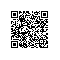# java数组的排序 对象数组的排序 Comoarable接口实现 compareTo函数实现

/*
java 中数组的排序 对象数组 的排序

java.until包中的 Arrays 类 的  binarySearch() sort()进行排序和 查找

String类 有 compareTo函数 可以直接进行比较 ,

*/
import java.util.Arrays; //排序函数在Arrays类中
class   Test
{
public static void main(String []args)
{
point []arr=new point[]{new point(12,"xiaoming"), new point(1,"xiaowang"),new point(4,"xiaoxiao"),new point(12,"xiaowei")};
Arrays.sort(arr);   //排序对象
for(int n=0;n<arr.length;n++)
{
System.out.println(arr[n]);   //对象直接回调用 toString函数 我们在类中重载了就会输出我们想要的信息
}

int index=Arrays.binarySearch(arr,arr);
System.out.println(index);
System.out.println("index element="+arr[index]);

}
}
class  point  implements Comparable  //实现Comparable接口  我们要实现 compareTo函数
{
int num;
String name;
point(int num,String name)
{
this.num=num;
this.name=name;
}
public int compareTo(Object o)   //重载compareTo函数
{
point p=(point)o;
int n= num>p.num?1:(num==p.num?0:-1);
if(n==0)
{
n=name.compareTo(p.name);
}
return n;
}
public   String toString()
{
return name+":"+num;
}
}使用钉钉扫一扫加入圈子
+ 订阅